Courses

# Properties of Inverse Trigonometric Functions Mathematics Notes | EduRev

## Mathematics : Properties of Inverse Trigonometric Functions Mathematics Notes | EduRev

The document Properties of Inverse Trigonometric Functions Mathematics Notes | EduRev is a part of the Mathematics Course Additional Topics for IIT JAM Mathematics.
All you need of Mathematics at this link: Mathematics

The inverse trigonometric functions are also called arcus functions or anti trigonometric functions. These are the inverse functions of the trigonometric functions with suitably restricted domains. Specifically, they are the inverse functions of the sine, cosine, tangent, cotangent, secant, and cosecant functions, and are used to obtain an angle from any of the angle’s trigonometric ratios.

Properties of Trigonometric Inverse Functions
Here are the properties of the inverse trigonometric functions with proof.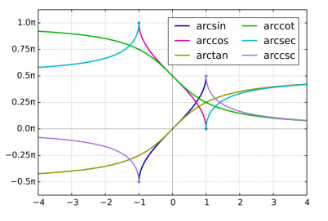Property 1
i. sin-1 (1/x) = cosec-1x , x ≥ 1 or x ≤ -1
ii. cos-1 (1/x) = sec-1x , x ≥ 1 or x ≤ -1
iii. tan-1 (1/x) = cot-1x , x > 0
Proof : sin-1 (1/x) = cosec-1x , x ≥ 1 or x ≤ -1,
Let  sin−1 x = y
i.e. x = cosec y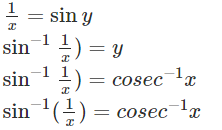Hence,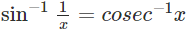where, x ≥ 1 or x ≤ -1.

Property 2
i. sin-1(-x) = – sin-1(x),    x ∈ [-1,1]
ii. tan-1(-x) = -tan-1(x),   x ∈ R
iii. cosec-1(-x) = -cosec-1(x), |x| ≥ 1
Proof: sin-1(-x) = -sin-1(x),    x ∈ [-1,1]
Let,  sin−1(−x) = y
Then −x = sin y
x = −sin y
x = sin(−y)
sin−1 = sin−1(sin(−y))
sin−1 x = y
sin−1 x = −sin−1(−x)
Hence,sin−1(−x)=−sin−1 x ∈ [-1,1]

Property 3
i. cos-1(-x) = π – cos-1 x, x ∈ [-1,1]
ii. sec-1(-x) = π – sec-1x, |x| ≥ 1
iii. cot-1(-x) = π – cot-1x, x ∈ R
Proof : cos-1(-x) = π – cos-1 x, x ∈ [-1,1]
Let cos−1(−x) = y
cos y = −x   x = −cos y
x = cos(π−y)
Since,  cosπ − q = −cos q
cos−1x = π − y
cos−1x = π–cos−1–x
Hence, cos−1−x = π–cos−1x

Property 4
i. sin-1x + cos-1x = π/2, x ∈ [-1,1]
ii. tan-1x + cot-1x = π/2, x ∈ R
iii. cosec-1x + sec-1x = π/2, |x| ≥ 1
Proof : sin-1x + cos-1x = π/2, x ∈ [-1,1]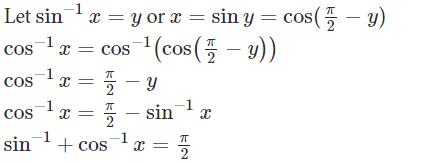Hence, sin-1x + cos-1x = π/2, x ∈ [-1,1]

Property 5
tan-1x + tan-1y = tan-1((x+y)/(1-xy)), xy < 1.
tan-1x – tan-1y = tan-1((x-y)/(1+xy)), xy > -1.
Proof : tan-1x + tan-1y = tan-1((x+y)/(1-xy)), xy < 1.
Let tan−1x = A
And tan−1y = B
Then, tan A = x
tan B = y
Now, tan(A+B)=(tanA+tanB)/(1−tanAtanB)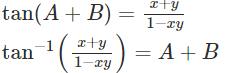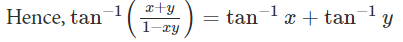Property 6
i. 2tan-1x = sin-1 (2x/(1+x2)), |x| ≤ 1
ii. 2tan-1x = cos-1((1-x2)/(1+x2)), x ≥ 0
iii. 2tan-1x = tan-1(2x/(1 – x2)), -1 < x <1
Proof : 2tan-1x = sin-1 (2x/(1+x2)), |x| ≤ 1
Let tan−1x = y and x = tan y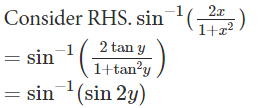Since, sin2θ = 2tanθ/(1+tan2θ),
= 2y
= 2tan−1x which is our LHS

Hence 2 tan-1x = sin-1 (2x/(1+x2)), |x| ≤ 1

Solved Example
Q1. Prove that “sin-1(-x) = – sin-1(x),    x ∈ [-1,1]”
Ans: Let, sin−1(−x) = y
Then −x = siny
x = −siny
x = sin(−y)
sin−1x = arcsin(sin(−y))
sin−1x = y
sin−1x = −sin−1(−x)
Hence, sin−1(−x)=−sin−1x, x ∈ [-1,1]

Offer running on EduRev: Apply code STAYHOME200 to get INR 200 off on our premium plan EduRev Infinity!

40 docs

,

,

,

,

,

,

,

,

,

,

,

,

,

,

,

,

,

,

,

,

,

;